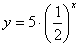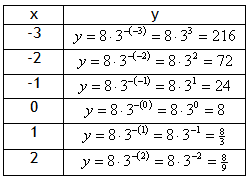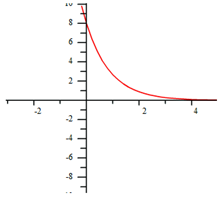Negative Reciprocal

Remember that a whole number to a negative power is equivalent to its reciprocal to the positive power. For instance, y = 5 · 2 ?x is equivalent to. Therefore, these types of functions also create exponential decay.

Example Graph the exponential function y = 8 · 3?x.

Step 1. Make a table of values.Step 2. Graph the function by plotting points.